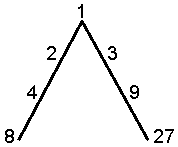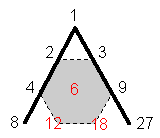<< Previous    1  2    4  5  ...14    Next >>

Plato's Lambda

In Timaeus, Plato’s treatise on Pythagorean cosmology, the central character, Timaeus of Locri (possibly a real person), describes how the Demiurge divided the World Soul into harmonic intervals. Having blended the three ingredients of the World Soul — Sameness, Difference and Existence — into a kind of malleable stuff, the Demiurge took a strip of it and divided its length into portions measured by the numbers forming two geometrical series of four terms each: 1, 2, 4, 8 and 1, 3, 9, 27, generated by multiplying 1 by 2 and 3 (Fig. 1). This became known as "Plato’s Lambda" because of its resemblance to Λ, the Greek letter lambda. Then, according to Timaeus:

he went on to fill up both the double and the triple intervals, cutting off yet more parts from the original mixture and placing them between the terms, so that within each interval there were two means, the one (harmonic)* exceeding the one extreme and being exceeded by the other by the same fraction of the extremes, the other (arithmetic) exceeding the one extreme by the same number whereby it was exceeded by the other. These links
gave rise to intervals of 3/2 and 4/3 and 9/8 within the original intervals. And he went on to fill up all the intervals of 4/3 (i.e., fourths) with the interval of 9/8 (the tone), leaving over in each a fraction. This remaining interval of the fraction had its terms in the numerical proportion of 256 to 243 (semitone). By this time, the mixture from which he was cutting off these portions was all used up.Figure 1. Plato's Lambda.

These numbers line but two sides of a tetractys array of ten numbers from whose relative proportions the musicians of ancient Greece worked out the frequencies of the notes of the now defunct Pythagorean musical scale.Figure 2. The Lambda Tetractys.

The three numbers missing from the Lambda are shown in red in Figure 2. The sum of the 10 integers is 90 and the sum of the integers 1, 8 & 27 at the corners of the tetractys is 36. The seven integers at the centre and corners of the grey hexagon shown in Figure 2 with dashed edges add up to 54. Hence, the 36:54 division displayed by the Lambda Tetractys differentiates between its corners, which correspond to the Supernal Triad of the Tree of Life, and its seven hexagonal yods, which correspond to the seven Sephiroth of Construction (see here). The division is shown by all holistic systems, as many of the articles on this website prove. Historians of science and musical scholars have focussed upon the integers as "number weights" whose significance is that their ratios are the tone ratios of the notes of the Pythagorean musical scale. They paid no attention to the magnitude of the numbers themselves because, influenced by the connotation given by Plato that their ratios determined the more important tone ratios, they seemed to have no relevance to music or anything else. The author's research into various sacred geometries and holistic systems indicates that this is untrue. The number 90 is always present in such systems as a defining parameter, as this section will demonstrate. The Lambda Tetractys has been regarded as nothing more than a heuristic device for generating the tone ratios of musical notes. Instead, it has profound, fundamental meaning, for — together with its tetrahedral generalisation to be described shortly — it characterizes the very nature of holistic systems. It is the arithmetic counterpart of the holistic pattern that sacred geometries embody.

* H is the harmonic mean of two positive numbers a and b (ba) if (b-H)/(H-a) = b/a. A is their arithmetic mean if (b-A)/(A-a) = 1.

 << Previous    1  2    4  5  ...14    Next >>

Home